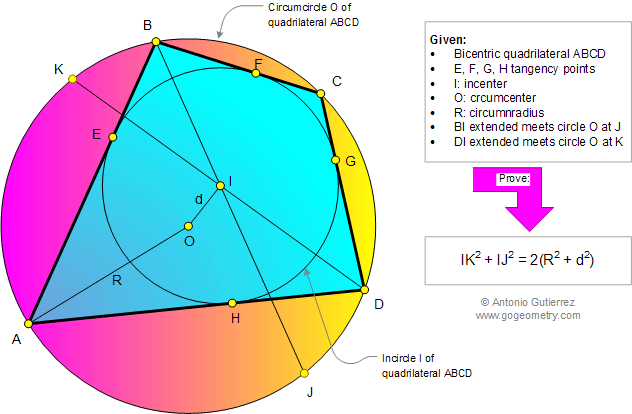# Online Geometry Problem 910: Bicentric Quadrilateral, Distance between the Incenter and Circumcenter, Incircle, Circumcircle, Circumscribed, Inscribed, Circumradius. Level: High School, College, Mathematics Education

< PREVIOUS PROBLEM  |  NEXT PROBLEM >

 In a bicentric quadrilateral ABCD, I is the incenter, O the circumcenter, R the circumradius, and IO = d. BI and DI extended meet the circumcircle O at J and K, respectively. Prove that IK2 + IJ2 = 2(R2 + d2). A bicentric quadrilateral ABCD is a convex quadrilateral that has both an incircle I and a circumcircle O.Home | Search | Geometry | Problems | All Problems | Open Problems | Visual Index | 10 Problems | Problems Art Gallery |  Art | 901-910 | Quadrilaterals | Cyclic Quadrilateral | Tangential or circumscribed quadrilateral | Circle Tangent Line | Solution / comment | by Antonio Gutierrez